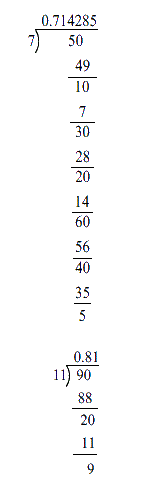In the verge of coronavirus pandemic, we are providing FREE access to our entire Online Curriculum to ensure Learning Doesn't STOP!

# Ex.1.3 Q8 Number System Solution - NCERT Maths Class 9

Go back to  'Ex.1.3'

## Question

Find three irrational numbers between the rational numbers \begin{align}\frac{5}{7}\end{align} and \begin{align}\,\frac{9}{{11}}\end{align}.

Video Solution
Number Systems
Ex 1.3 | Question 8

## Text Solution

Steps:

Let us find the decimal expansion of \begin{align}\frac{5}{7}\end{align} and \begin{align}\frac{9}{{11}}\end{align} .We can write $$3$$ irrational numbers between them as follows:

(i) \begin{align}0.723350980011234...\end{align}

(ii) \begin{align} 0.77653472899423…\end{align}

(iii) \begin{align}0.80983435253947…\end{align}

Video Solution
Number Systems
Ex 1.3 | Question 8

Learn from the best math teachers and top your exams

• Live one on one classroom and doubt clearing
• Practice worksheets in and after class for conceptual clarity
• Personalized curriculum to keep up with school